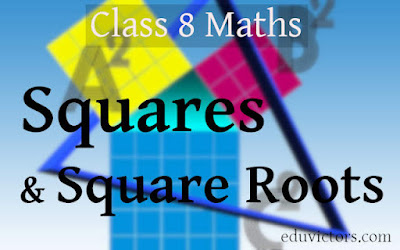## Pages

Showing posts with label imo-class9. Show all posts
Showing posts with label imo-class9. Show all posts

## Wednesday, 7 November 2012

### Class 9 - Maths - PSA Sample Test Paper/NTSE MAT Quiz-16

Class 9 - Maths - PSA Sample Test Paper
NTSE MAT Quiz-16
(Questions asked in one of  the public school at Delhi. PSA stands for Problem Solving Assessment, a test to be conducted by schools as per CBSE guidelines)

MCQs

Q1: If Rekha can type a page in 'm' minutes, what piece of the page she can do it in 10 minutes?

(a) 10/m
(b) m - 10
(c) M + 10
(d) m/10## Wednesday, 25 July 2012

### Class 9 - Maths - History of Indian Mathematics - MCQsAryabhatta - statute at University of Astrophysics at Puneimage credits: wikipedia
History of Indian Mathematics - MCQs
(Questions taken from MP Board Question bank for class 9.)
(Answers are provided at the end)

Q1: Main branch of Mathematics is :
(a) Arithmetic
(b) Algebra
(c) Geometry
(d) Accountancy

Q2: Which is the base of scientific development?
(a) Physics
(b) Chemistry
(c) Mathematics
(d) None of these## Sunday, 8 July 2012

### NTSE SAT Quiz-13 (Maths) / Class 8 Maths

NTSE SAT Quiz-13 (Maths)

Q1: One third of a number is greater then one fourth of its successor by 1, find the number.

(a) 5
(b) 15
(c) 20
(d) 25## Coordinate Geometry - Important Points

1. Each point on a number line is associated with a number called its coordinate.

2. Each point on a plane is associated with an ordered pair (x,y) of numbers called its coordinates.# Class 8, NTSE - Maths - Square and Square Roots

### Perfect Square

1. A number is called a perfect square if it is expressed as the square of a number.
2. E.g. 1, 4, 9, 16, 25, ... are called perfect squares (1x1 = 1, 2x2 = 4, 3 x 3 = 9...)
3. In square numbers, the digits at the unit’s place are always 0, 1, 4, 5, 6 or 9.
4. The numbers having 2, 3, 7 or 8 at their units' place are not perfect square numbers.

Q1: Which one of the following numbers is a perfect square:
a) 622
b) 393
c) 5778
d) 625

5. If a number ends with the odd number of zeros then it is not a perfect square.

Q2: Check which of the following is not a perfect square.
a) 81000
b) 8100
c) 900
d) 6250000

Answer: a) 81000 (= 92 x 102 x 10)

6. The square of an even number is an even number while the square of an odd number is an odd number.

7. If n is a positive whole number then (n+1)2 - n2 = 2n + 1
or 2n numbers in between the squares of the numbers n and (n + 1)## Tuesday, 15 November 2011

### NTSE MAT Quiz-1 (Series completion)

Complete the following series:

1.  6,12,21,___,48,66
(a) 33      (b) 38       (c) 40       (d) 45

2.  125,80,45,20,___
(a)  5        (b)    8     (c) 10        (d)12

3.  22,24,28,___,52,84

(a)  30        (b)   36      (c)    42     (d) 46

4. 1,3,3,7,6,13,10,___,15,31

(a)  25        (b)   27      (c)    21     (d) 30

5. 3,1,7,3,13,7,21,15,31,31,___,63,57

(a)    39      (b) 41        (c)  38       (d) 43